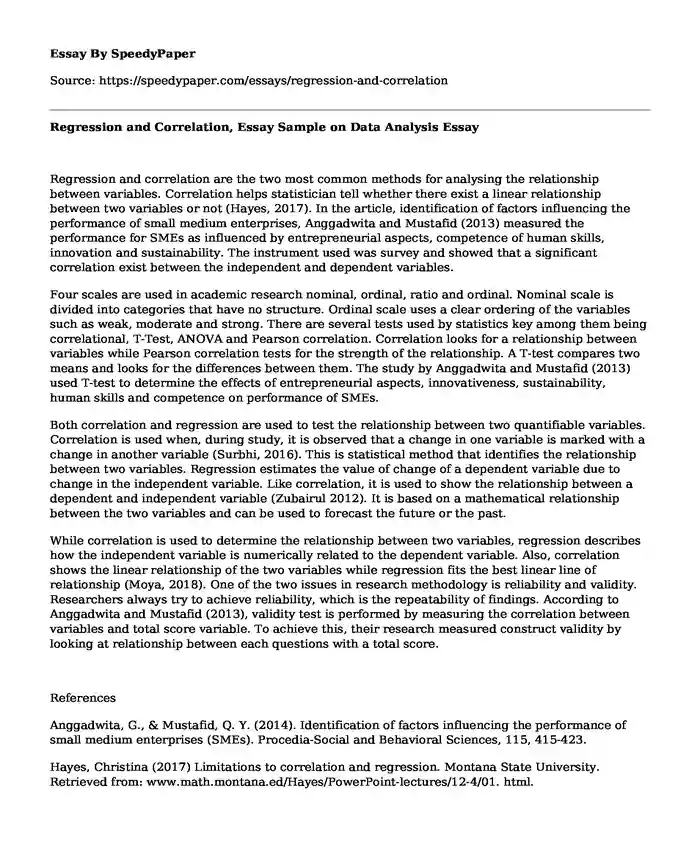# Regression and Correlation, Essay Sample on Data Analysis

Published: 2022-03-11Type of paper: Essay Categories: Data analysis Pages: 2 Wordcount: 473 words
143 views

Regression and correlation are the two most common methods for analysing the relationship between variables. Correlation helps statistician tell whether there exist a linear relationship between two variables or not (Hayes, 2017). In the article, identification of factors influencing the performance of small medium enterprises, Anggadwita and Mustafid (2013) measured the performance for SMEs as influenced by entrepreneurial aspects, competence of human skills, innovation and sustainability. The instrument used was survey and showed that a significant correlation exist between the independent and dependent variables.Is your time best spent reading someone else’s essay? Get a 100% original essay FROM A CERTIFIED WRITER!

Four scales are used in academic research nominal, ordinal, ratio and ordinal. Nominal scale is divided into categories that have no structure. Ordinal scale uses a clear ordering of the variables such as weak, moderate and strong. There are several tests used by statistics key among them being correlational, T-Test, ANOVA and Pearson correlation. Correlation looks for a relationship between variables while Pearson correlation tests for the strength of the relationship. A T-test compares two means and looks for the differences between them. The study by Anggadwita and Mustafid (2013) used T-test to determine the effects of entrepreneurial aspects, innovativeness, sustainability, human skills and competence on performance of SMEs.

Both correlation and regression are used to test the relationship between two quantifiable variables. Correlation is used when, during study, it is observed that a change in one variable is marked with a change in another variable (Surbhi, 2016). This is statistical method that identifies the relationship between two variables. Regression estimates the value of change of a dependent variable due to change in the independent variable. Like correlation, it is used to show the relationship between a dependent and independent variable (Zubairul 2012). It is based on a mathematical relationship between the two variables and can be used to forecast the future or the past.

While correlation is used to determine the relationship between two variables, regression describes how the independent variable is numerically related to the dependent variable. Also, correlation shows the linear relationship of the two variables while regression fits the best linear line of relationship (Moya, 2018). One of the two issues in research methodology is reliability and validity. Researchers always try to achieve reliability, which is the repeatability of findings. According to Anggadwita and Mustafid (2013), validity test is performed by measuring the correlation between variables and total score variable. To achieve this, their research measured construct validity by looking at relationship between each questions with a total score.

References

Anggadwita, G., & Mustafid, Q. Y. (2014). Identification of factors influencing the performance of small medium enterprises (SMEs). Procedia-Social and Behavioral Sciences, 115, 415-423.

Hayes, Christina (2017) Limitations to correlation and regression. Montana State University. Retrieved from: www.math.montana.ed/Hayes/PowerPoint-lectures/12-4/01. html.

Mason, Moya (2018). Correlation and Regression Bivariate analysis. , Canada.

Surbhi, S (2016). Differences between correlation and regression. Retrieved from https://keydifferences.com/differences-between-correlation-and-regression-htmlZubairul, Islam (2012).Compare and contrast correlation with regression.

Regression and Correlation, Essay Sample on Data Analysis. (2022, Mar 11). Retrieved from https://speedypaper.com/essays/regression-and-correlation

Request Removal

If you are the original author of this essay and no longer wish to have it published on the SpeedyPaper website, please click below to request its removal:

Liked this essay sample but need an original one?

Hire a professional with VAST experience!